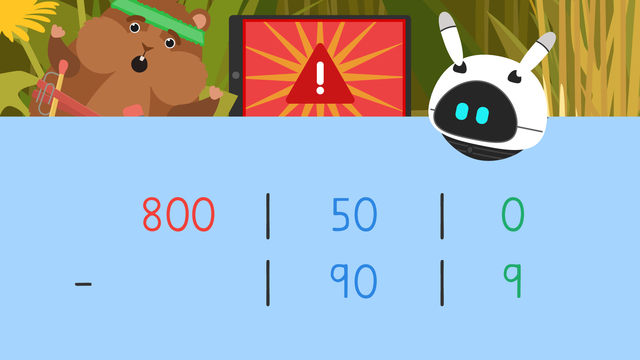# Three Digit Subtraction with Regrouping - Expanded FormRating

Ø 5.0 / 2 ratings

The authorsTeam Digital
Three Digit Subtraction with Regrouping - Expanded Form
CCSS.MATH.CONTENT.3.NBT.A.2

## Basics on the topicThree Digit Subtraction with Regrouping - Expanded Form

### In this 3rd Grade Video on Expanded Form Subtraction with Regrouping

Mr. Squeaks and Imani have been playing a lot of games on their devices lately and now they can’t get any to load! Did they each use up all their data already? In this video, Mr. Squeaks and Imani learn about three digit subtraction with regrouping using expanded form to determine how data is left.

### 3 Digit Subtraction Using Expanded Form

Expanded form is a way to write a number that emphasizes the value each digit holds. In a subtraction equation, we can write each number in expanded form to make regrouping easier.Let’s use the example eight hundred fifty minus ninety-nine to model our steps. First, write eight hundred fifty and ninety-nine in expanded form with the larger number on top. We'll put separation lines in place of the addition signs, so we don't confuse ourselves as we subtract.Then, we subtract the ones column: zero minus nine. Since we cannot subtract from zero, we will regroup a ten from the tens place. Now, we subtract ten minus nine. Write the difference, one, below.Then, we subtract the tens column, forty minus ninety. Since there is not enough to subtract from, we regroup one hundred from the hundreds place. We can now subtract: one hundred forty minus ninety. Write the difference, fifty, below.Next, we subtract the hundreds column: seven hundred minus zero. We write the difference, seven hundred, below. Finally, put the answer back in standard form by adding it together. Seven hundred plus fifty plus one becomes seven hundred fifty-one.### Summary of Steps: 3 Digit Subtraction Using Expanded Form

Remember, we can write each number in a subtraction equation using expanded form to make regrouping easier.

• First, subtract the ones column. If there is not enough to subtract from, regroup a ten from the tens place and subtract.

• Then, move to the tens and the hundreds place repeating this process as necessary.

• Finally, put the answer back in standard form by adding it together.

Have you practiced yet? On this website, you can also find 3 digit subtraction expanded form worksheets and exercises.

### TranscriptThree Digit Subtraction with Regrouping - Expanded Form

Mr. Squeaks and Imani are ready to play on their devices, but nothing will load! "Did we run out of data, Imani?" "Let's check our devices to see if there's any left." However, they are having trouble subtracting from three digit numbers. Let's help Imani and Mr. Squeaks solve their equations by learning "Three Digit Subtraction with Regrouping - Expanded Form". Expanded form is a way to write a number that emphasizes the value each digit holds. In a subtraction equation, we can write each number in expanded form to make regrouping easier. Let's check Mr. Squeaks data usage to practice. It says he has eight hundred fifty megabytes of data and used ninety-nine, how much data is left? First, write eight hundred fifty and ninety-nine in expanded form with the larger number on top. We'll put separation lines in place of the addition signs, so we don't confuse ourselves as we subtract. Then, subtract the ones column: zero minus nine. Since there is not enough to subtract from, regroup a ten from the tens place. Now subtract ten minus nine. Write the difference, one, below. Then, subtract the tens column, forty minus ninety. Since there is not enough to subtract from, regroup one hundred from the hundreds place and subtract, one hundred forty minus ninety. Write the difference, fifty, below. Subtract the hundreds column, seven hundred minus zero, and write the difference, seven hundred, below. Finally, put the answer back in standard form by adding it together, seven hundred fifty-one. Huh, Mr. Squeaks should have seven hundred fifty one megabytes of data left. Now, Imani checks their data usage. It says Imani has three hundred megabytes of data and used two hundred ninety-nine..how much data is left? What should we do first? First, write three hundred minus two hundred ninety-nine in expanded form like this. Do you know our next step? Next, subtract the ones column, but we cannot take nine away from zero so regroup a ten from the tens place and subtract, but there is nothing we can borrow since there is zero there. Where can we regroup from? We can regroup one hundred from the hundreds column. Now that there is a hundred to borrow from in the tens place, we can take a ten to regroup for the ones place. Where should we subtract now? The ones place. Ten minus nine is one and we write the difference, here. Then, we move to the tens place and subtract ninety from nine to get zero. Finally, subtract the hundreds place: two hundred minus two hundred equals zero. What is our very last step? Put the answer back in standard form by adding it together, one! Huh, Imani still has one megabyte of data left! Before we solve the data dilemma, let's summarize! Remember, we can write each number in a subtraction equation using expanded form to make regrouping easier. First, subtract the ones column. If there is not enough to subtract from, regroup a ten from the tens place and subtract. Then, move to the tens and the hundreds place repeating this process as necessary. Finally, put the answer back in standard form by adding it together. "I just don't get it, Imani we should both have enough data left to keep playing." "Umm, Mr. Squeaks, I think I forgot to unplug my bed… and plug in the wifi."